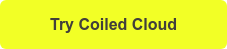# Converting a Dask DataFrame to a pandas DataFrame

October 1, 2021

This post explains how to convert from a Dask DataFrame to a pandas DataFrame and when it’s a good idea to perform this operation.

Each partition in a Dask DataFrame is a pandas DataFrame. Converting from a Dask DataFrame to a pandas DataFrame combines multiple pandas DataFrames (partitions) into a single pandas DataFrame.

Dask DataFrames can store massive datasets, whereas pandas DataFrames must be smaller than the memory of a single computer. This means only small Dask DataFrames can be converted into pandas DataFrames.

This post shows the syntax to perform the conversion, the error message if the Dask DataFrame is too big, and how to assess if conversion is possible.

Let’s dive in.

## Convert from Dask to pandas on localhost

Start by creating a Dask DataFrame. All computations are in this notebook.

```import dask.dataframe as dd
import pandas as pd

df = pd.DataFrame(
{"nums": [1, 2, 3, 4, 5, 6], "letters": ["a", "b", "c", "d", "e", "f"]}
)
ddf = dd.from_pandas(df, npartitions=2)```

Now convert the Dask DataFrame into a pandas DataFrame.

`pandas_df = ddf.compute()`

`type(pandas_df)` returns `pandas.core.frame.DataFrame`, which confirms it’s a pandas DataFrame.

You can also print `pandas_df` to visually inspect the DataFrame contents.

```print(pandas_df)

nums letters
0     1       a
1     2       b
2     3       c
3     4       d
4     5       e
5     6       f```

We’re easily able to convert a small Dask DataFrame to a pandas DataFrame on localhost (your local computer). Let’s try to convert a big Dask DataFrame to a pandas DataFrame on a cloud-based cluster.

## Converting big datasets on the cloud

Create a 5 node Dask cluster with Coiled and read in 662 million rows of time series data into a Dask DataFrame.

```import coiled

cluster = coiled.Cluster(name="demo-cluster", n_workers=5)

"s3://coiled-datasets/timeseries/20-years/parquet",
storage_options={"anon": True, "use_ssl": True},
engine="pyarrow",
)```

`ddf` contains 662 million records and uses 58 GB of memory.  We won’t be able to convert this whole Dask DataFrame to a Pandas DataFrame because it’s too big.  Let’s run a filtering operation and see if a subset of the Dask DataFrame can be converted to a pandas DataFrame.

`filtered_ddf = ddf.loc[ddf["id"] > 1150]`

`filtered_ddf` contains 1,103 rows of data and only uses 102 KB of memory.  It’s a tiny subset of the original Dask DataFrame that contains 662 million rows.

This filtered dataset can easily be converted to a Pandas DataFrame with `filtered_ddf.compute()`.

See this blog post on filtering DataFrames and this blog post on computing memory usage of DataFrames for more background information on these topics.

Just for fun, let’s try to convert the entire Dask DataFrame to Pandas and see what happens.

## Error when converting a big dataset

The entire Dask DataFrame takes 58 GB of memory, and we already know that’s too much data to be collected into a single Pandas DataFrame.The nodes in our cluster only have 16 GB of memory. You’d need a Dask DataFrame that’s smaller than the memory of a single node to collect the data in a Pandas DataFrame.

`ddf.compute()` will error out.

Even if the Dask DataFrame is sufficiently small to be converted to a pandas DataFrame, you don’t necessarily want to perform the operation.

## When to convert to pandas

Dask uses multiple cores to operate on datasets in parallel. Dask is often quicker than pandas, even for localhost workflows, because it uses all the cores of your machine.

You may want to convert to a pandas DataFrame to utilize libraries like scikit-learn or matplotlib.

For one-off tasks, it’s fine reverting to pandas, but it should generally be avoided when building production data pipelines. pandas cannot scale when data sizes grow. Data pipelines that can scale up are more robust. Rebuilding large components of systems when datasets grow isn’t ideal.

Only convert to pandas for one-off tasks or when you’re sure datasets will stay small and you won’t have to deal with pandas scaling issues in the future.

## Conclusion

It’s easy to convert a Dask DataFrame to a pandas DataFrame when the dataset is small.

If the dataset is big, this operation will error out.

You’ll generally want to avoid converting Dask DataFrame to pandas DataFrames because you’ll lose out on all the benefits of Dask. Dask offers parallel computing, which is more flexible because it can be scaled up when data sizes grow.

Thanks for reading! And if you’re interested in trying out Coiled Cloud, which provides hosted Dask clusters, docker-less managed software, and one-click deployments, you can do so for free today when you click below.